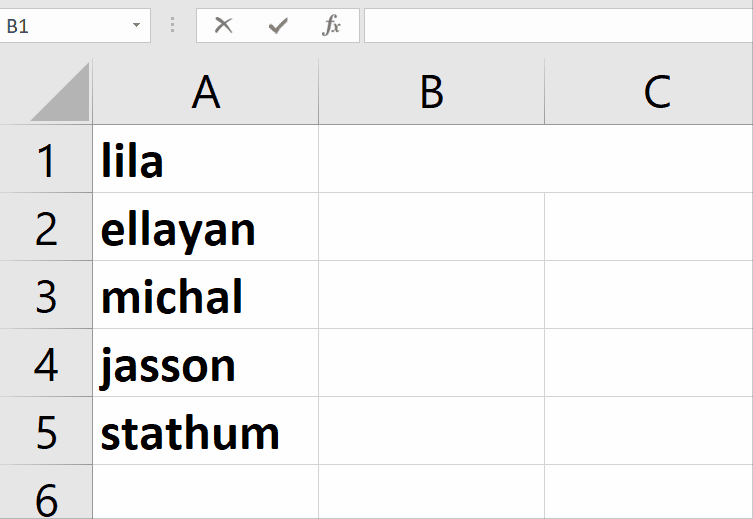# How to capitalize first letters in excel

During typing in Excel, users face difficulty in changing the case of words.

In this tutorial we will learn how to capitalize the first letter in Excel. To handle this issue, Microsoft Excel has a built in function

=PROPER(text)

In the formula, between the parenthesis, there is a text place, here will be the name of the cell containing our desired word, to capitalize the first letter. Let’s start with an example;Excel offers incredible features to ease mathematical calculations by using built-in formulas. Like Microsoft Word, Excel does not provide any hotkey to change the case of written words.

### Step 1 – Apply formula– Select a blank cell, in which you would like to change the case of a word.

– In this case, the selected cell is B2, and the cell to be converted is A1.

– The formula would be =PROPER(A1).

– As soon as you press the enter key, Excel will change the formatting from lower case to proper case.

Above is an animation as example.